Xu, L., Zhou, J., Ni, J., Li, Y., Long, Y., and Huang, R. (2020). "Investigating the pyrolysis kinetics of Pinus sylvestris using thermogravimetric analysis," BioRes. 15(3), 5577-5592.

#### Abstract

Thermogravimetric analyses of Pinus sylvestris from Xinxiang were performed to investigate its kinetic characteristics, which could provide information for industrial applications. Thermal degradation experiments were conducted at various heating rates of 10 °C/min, 20 °C/min, and 60 °C/min using a thermogravimetric analysis-differential scanning calorimetry (TG-DSC) analyzer with an inert environment. The peak pyrolysis temperatures of the three major components (hemicellulose, cellulose, and lignin) were predicted by the Kissinger-Kai method, and activation energy values (Eα) were calculated. The Eα of Pinus sylvestris was also estimated by two model-free methods. The decomposition reactions of hemicellulose, cellulose, and lignin at different temperatures were the main reason for fluctuations in Eα. The time for heat transfer was less sufficient at a high heating rate compared with that at a low heating rate, which caused the temperature gradients in the samples. Therefore, the temperature of maximum exothermic peaks was higher than the maximum pyrolysis temperature. This kinetic study could be useful for providing guidance for optimizing the biomass pyrolysis process.

Investigating the Pyrolysis Kinetics of Pinus sylvestris Using Thermogravimetric Analysis

Langui Xu,a Jiawei Zhou,a Jiong Ni,a Yanru Li,b Yan Long,c and Ruyi Huang a,c,d,*

Thermogravimetric analyses of Pinus sylvestris from Xinxiang were performed to investigate its kinetic characteristics, which could provide information for industrial applications. Thermal degradation experiments were conducted at various heating rates of 10 °C/min, 20 °C/min, and 60 °C/min using a thermogravimetric analysis-differential scanning calorimetry (TG-DSC) analyzer with an inert environment. The peak pyrolysis temperatures of the three major components (hemicellulose, cellulose, and lignin) were predicted by the Kissinger-Kai method, and activation energy values (Eα) were calculated. The Eα of Pinus sylvestris was also estimated by two model-free methods. The decomposition reactions of hemicellulose, cellulose, and lignin at different temperatures were the main reason for fluctuations in Eα. The time for heat transfer was less sufficient at a high heating rate compared with that at a low heating rate, which caused the temperature gradients in the samples. Therefore, the temperature of maximum exothermic peaks was higher than the maximum pyrolysis temperature. This kinetic study could be useful for providing guidance for optimizing the biomass pyrolysis process.

Keywords: Pinus sylvestris; Pyrolysis; Thermogravimetry; Activation energy

Contact information: a: School of Mechanical Engineering, North China University of Water Resources and Electric Power, Zhengzhou 450011, China; b: College of Architecture and Urban-Rural Planning, Sichuan Agricultural University, Chengdu 611830, China; c: Biogas Institute of Ministry of Agriculture, Risk Assessment Lab of the Quality Safety of Biomass Fermentation Products (Chengdu), Ministry of Agriculture and Rural Affairs, Chengdu 610041 China; d: Rural Energy Office of Sichuan Province, Chengdu, 610041 China; *Corresponding author: huangruyi1983@qq.com

INTRODUCTION

The world’s energy demand continues to grow with economic development and population growth, although energy efficiency is on the rise. The consumption of fossil fuels induces global climate change, and sources of fossil fuel energy are dwindling (Huang et al. 2019). Renewable energy has been beneficial for solving the energy shortage of countries. Biomass energy, which can be converted into solid, liquid, and gaseous fuels, is a unique renewable energy and renewable carbon source (Nurek et al. 2019). Biomass energy, such as that derived from agricultural and forestry residues, is abundant and considered to be the fourth largest energy resource (Cai et al. 2019; Rodríguez-Jiménez et al. 2019).

Biomass fuels can be roughly divided into three categories: wood and forestry processing residues, herbs, and agricultural processing residues. With its high forest coverage, China is considered an agricultural country. Thus, the annual production of agricultural and forest residues is vast. Agricultural and forest residues are collectively referred to as lignocellulosic biomass and are effective in generating heat and power via thermochemical processes (Ding et al. 2016). Forest biomass contains fewer harmful elements, and there is little slagging and exhaust emission after combustion, which is the main superior quality of bioenergy (Wu et al. 2019; Ding et al. 2020). Pinus sylvestris, which can release more heat than other wood, is a typical and flammable plant with a large amount of turpentine. This plant burns completely and is environmentally friendly. Due to its physical properties, Pinus sylvestris is one of the most popular biomass energy sources.

Characterization of biomass determines its fuel potential. The best energy input utilization can be obtained by studying the thermal characteristics and fundamental chemistry of biomass. Pyrolysis processes are the first step in gasification and combustion, which is the thermal conversion reaction in which biomass transforms into chars, gases, and liquids of oxygenated organic compounds (Wang et al. 2017). Many researchers have studied the biomass pyrolysis characteristics of Lentinus edodes (Zou et al. 2019), cattle manure (Zhang et al. 2019), incense sticks (Wen et al. 2019), sorghum straw (Dhyani et al. 2017), coffee ground residues (Fermoso and Mašek 2018), corn stalk (Cai et al. 2018), and so on. However, the properties of biomass can vary in different environments and climates.

The northern part of Xinxiang, Henan Province of China, with its rich forest resources, is located in the hilly land of the Taihang Mountains. Pinus sylvestris is planted in large areas. This paper studied the thermochemical processes of Pinus sylvestris from Xinxiang. The results could provide guidance for optimizing equipment. The Pinus sylvestris samples were subjected to thermogravimetric analysis with different heating rates (10 °C/min, 20 °C/min, and 60 °C/min) to determine its characteristics. The nonisothermal kinetic methods of Kissenger-Akahira-Sunose (KAS) and Flynn-Wall-Ozawa (FWO) were used to determine pyrolysis kinetics to preferentially describe the thermal decomposition process. These methods were efficient in calculating activation energy values (Eα) because there was no reaction model assumption (Vyazovkin et al. 2011; Shi et al. 2017). Using experimental data, the peak pyrolysis temperatures of the three major components (hemicellulose, cellulose, and lignin) were predicted by the Kissinger-Kai method, and Eα values were also calculated in this paper.

EXPERIMENTAL

Materials

Pinus sylvestris used in this study was sampled from the countryside of Xinxiang, China. The samples were dried at ambient atmosphere for 3 d to remove their moisture, and then they were crushed and sieved through an 80-mesh screen to obtain a uniform size, which could lower temperature gradient within particles. The samples were then placed in plastic bags to prevent moisture absorption.

Methods

Proximate and ultimate analyses

An automatic proximate analyzer (5E-MAC/GIII; Changsha Kaiyuan Instruments Co., Ltd., Changsha, China) was used to perform proximate analysis to determine volatile matter (VM), moisture (M), and ash (A) contents. Fixed carbon (FC) was determined by the equation: FC = 100 – (M + A + VM). An ultimate analyzer with a Vario EL cube (Elementar, Langenselbold, Germany) was used to estimate the contents of hydrogen (H), carbon (C), sulfur (S), nitrogen (N), and oxygen (O calculated by difference). All results are presented in Table 1.

Table 1. Proximate and Ultimate Analyses of Pinus sylvestris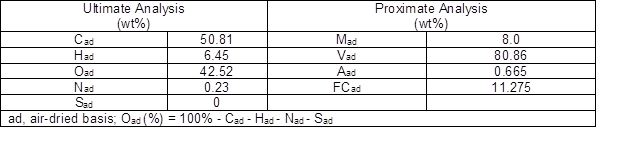Pyrolysis tests

Thermogravimetric (TG) analysis was performed with a TG analyzer (SDT Q600; TA Instruments, New Castle, DE, USA) in N2 atmosphere. Samples with weights lower than 8 mg were placed in alumina crucibles and then heated from room temperature to 900 °C at heating rates of 10 °C/min, 20 °C/min, and 60 °C/min under a nitrogen gas flow rate at 100.0 mL/min. Each experiment was repeated twice to ensure repeatability.

Mathematical Model Development

The TG supplied a quality atmosphere and an alterable heating rate, which reduced the effect of thermal gradients and heat transport during pyrogenic decomposition of small samples (Jiang et al. 2015). A mathematical model was developed to analyze the TGA-differential scanning calorimetry (DSC) data. The rate of material decomposition with the iso-conversional method is given as Eq. 1,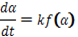(1)

where á is the conversion rate, which is defined in Eq. 2,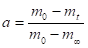(2)

where m0 is the initial mass (mg), mt is the actual mass (mg) at t moment, and m is the final residual mass (mg).

The reaction model is represented by f(á), which is a function of the conversion rate as shown in Eq. 3,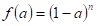(3)

where n is the reaction order and k is the constant of the reaction rate, which is dependent on the temperature expressed by the Arrhenius equation, which is shown in Eq. 4,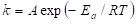(4)

where Eα is activation energy value (kJ/mol), T is absolute pyrolysis temperature (K), R is the universal gas constant (8.31 J/(K·mol)), and is the pre-exponential factor (s-1).

Introducing the heating rate, â (K/min), shown in Eq. 5,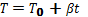(5)

and combining Eq. 1 and Eq. 4 with Eq. 5 results in Eq. 6 below: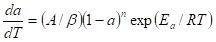(6)

Equation 6 was integrated after rearrangement with the conversion rate from zero, so the total conversion can be given as follows in Eq. 7,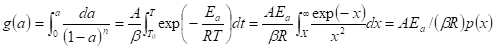(7)

where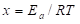,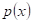is the temperature integral without the analytical solution and g(a) is the conversion quantity from the start of the experiment to the moment t. The methods used to evaluate kinetic parameters from TGA data are based on Eqs. 6 and 7.

Kinetic Analysis

The kinetic parameters of nonisothermal thermal experiments were estimated to optimize the process of biomass thermal degradation. The KAS and FWO methods were used to calculate kinetic and thermodynamic parameters of the samples without model assumption.

KAS method

The KAS method, which is expanded from Kissinger (1957) and Akahira and Sunose (1971), is a model-free method that is expressed as follows in Eq. 8: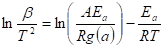(8)

For a given conversion rate, the line of ln (β/T2) against 1/was plotted, and the Eá could be calculated by the slope expression E/R. If g(α) could be determined, the pre-exponential factor A (s-1) was also calculated by the intercept from Eq. 8.

FWO method

The FWO method derived from the Doyle approximation is another model-free model that is often used to estimate kinetic parameters. The FWO method can be expressed as shown in Eq. 9,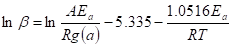(9)

where g(α) is expressed as in Eq. 7. Because ln[(AE)/(Rg(α))] did not relate to β, the Eá was determined using the slope expression (-1.0516 Ea R). There was no assumption concerning the reaction mechanism for the Ea calculation. The pre-exponential factor A could be obtained from the intercept expression ln[(AE)/(Rg(α))] when g(α) was determined.

Kissinger-Kai method

The Kissinger-Kai method, based on the Kissinger method (Kissinger 1956), was developed by Li et al. (2014). Hemicellulose, cellulose, and lignin are the main components of lignocellulosic biomass, and each component should decompose at different peak temperatures. The corresponding activation energies can be computed when peak values are presented. The absolute second derivative of TG (|DDTG|) can determine the peak locations corresponding to the main components, as shown in Eq. 10,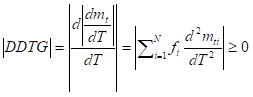(10)

where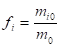is the initial mass fraction of every part i, and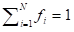. When one component reached the maximum decomposition rate at the peak temperature,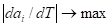at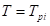, its second derivative (|DDTG|) would decrease rapidly to a local minimum (Channiwala and Parikh 2002). The peak temperature of main components (Tp,i ) could be confirmed by the minimum value if there were no other major peaks nearby. Then, Eα was confirmed by the Kissinger method for different peaks, as shown in Eq. 11,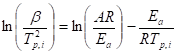(11)

where p,i denotes the peak temperature (°C) of different components, and Eα can be calculated by the slope expressions,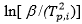against (1/Tp,i ).

RESULTS AND DISCUSSION

Physicochemical Properties of Pinus sylvestris

Moisture content of the samples reached 8.0% as shown in the proximate analysis results in Table 1. The samples were considered quality fuels because moisture content was less than 10%, which would be suitable for combustion (Font et al. 2005). Volatile matter increased to 80.1%, and ash content decreased to 0.665%. Because of low ash content, Pinus sylvestris samples were not easy to slag when burned (Chen et al. 2019). The samples contained no sulfur and little nitrogen, which caused few emissions of toxic gases during pyrolysis and combustion. High heating values (HHV, MJ/kg) of the samples, which is the total energy released when subjected to combustion, can be calculated with Eq. 12 (Nhuchhen and Abdul Salam 2012),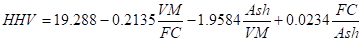(12)

where VM is volatile matter (%) and FC is fixed carbon (%), and the HHV of Pinus sylvestris was calculated as 18.16 MJ/kg.

Influence of Heating Rate on TG and DTG Analyses

Thermogravimetry ((D)TG) analyses were used to study thermal conversion of the samples. Weight of residual mass of the samples varied with pyrolysis temperature. Water evaporation, hemicellulose, cellulose, and lignin gradually decomposed with the increase in temperature. Total biomass decomposition could be considered as the superposition of these main components (Ahmad et al. 2017). The TG experiment was conducted at heating rates of 10 °C/min, 20 °C/min, and 60 °C/min from ambient temperature to 900 °C at a N2 flow rate of 100 mL/min. Figure 1 shows the effect of heating rate on Pinus sylvestris mass loss rate. With the increase in heating rate (β), TG and DTG curves moved to higher temperature regions. However, the thermal decomposition trend was not affected. The temperature transformation showed the thermal hysteresis. Therefore, with the increase in heating rate, atmosphere temperature in the TG analyzer increased during the time interval when heat transferred to the sample center.. As the heating rate decreased, the mass losses increased as the reaction became more sufficient. Similar conclusions were also reported in other studies (Ding et al. 2016; Ahmad et al. 2017).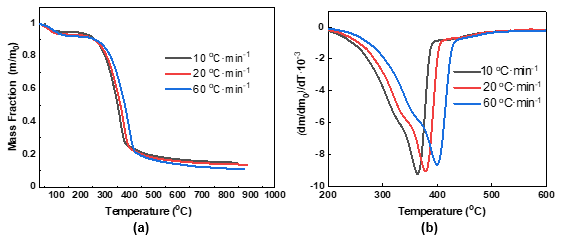Fig. 1. TG (a) and DTG (b) curves of Pinus sylvestris as a function of temperature in a N2 atmosphere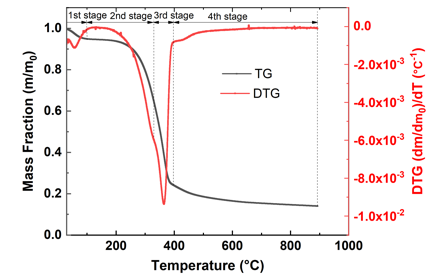Fig. 2. TG/DTG curves of Pinus sylvestris at a heating rate of 10 °C/min

The three-main components of lignocellulosic biomass have different structure. Hemicellulose has an amorphous structure with little strength which is easy to pyrolyze at low temperatures. Cellulose is crystalline and has strong resistance to hydrolysis with a high pyrolysis temperature range. Lignin is heavily interconnected, filling the three-dimensional space of biomass with a wide pyrolysis temperature range. Therefore, there were four different mass loss stages in Fig. 2. For the heating rate of 10 °C/min, the moisture was free water in the sample (surface moisture and inherent moisture) that evaporated from room temperature to 105 °C in the first stage, and the total mass loss was approximately 5%. At the second stage, mass loss was mainly because hemicellulose decomposed. Total mass loss was approximately 48% from 105 °C to 330 °C. At the third stage, a maximum mass loss occurred because cellulose decomposed, and total mass loss reached 76% between 320 °C and 397 °C. The total mass loss percent could reach approximately 84% from 397 °C to 900 °C, which was attributed to lignin degradation (Lahijani et al. 2019). There was a rapid decrease in mass loss rate until 397 °C. Then, there was a slow decrease in mass loss rate, and mass change was small after 550 °C.

DSC and Heat Flow Measurement

Variation in heat flow (mW/mg) of the samples with change in temperature was studied, and the DSC graphs in Fig. 3 show heating flow variations at different heating rates.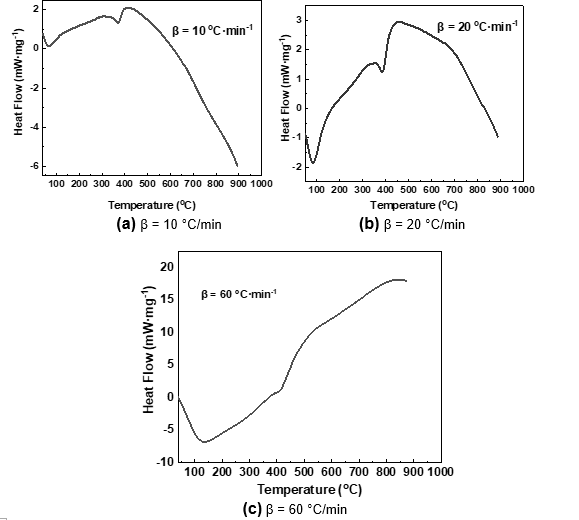Fig. 3. DSC curves of Pinus sylvestris at different heating rates

The heat flow had a nonlinear nature, as shown in Fig. 3. The endothermic peak in all heating rates was approximately 100 °C because moisture was removed from the samples. Exothermic reactions began during pyrolysis. A rapid increase in heat flux was observed as temperature increased from 200 °C to 400 °C. At approximately 400 °C, a second endothermic peak appeared in each of the three heating rates because the crystal water was separated. At this point, pyrolysis was still an exothermic reaction. Maximum heat flow increased with the increase in heating rate, which could reach 405 °C, 455 °C, and 830 °C with heating rates of 10 °C/min, 20 °C/min, and 60 °C/min, respectively. Maximum heat flow was obtained at a higher temperature than the max pyrolysis temperature in DTG, which was 364 °C at 10 °C/min, 378 °C at 20 °C/min, and 399 °C at 60 °C/min, as shown in Fig. 2. This result indicated that the hysteresis effect existed between inner and outer parts of the sample, which hindered the release of heat flow (Huang et al. 2018).

After heat flux temperatures reached a maximum, heat flow decreased because the reaction became weak, as shown in Fig. 2. The heat flow decreased with the decrease in mass change until completion of the reaction.

KINETIC PARAMETERS ANALYSES

Activation Energies of Hemicellulose, Cellulose, and Lignin

The order of thermal degradation of the three main substances from easiest to most difficult was hemicellulose > cellulose > lignin (Grønli et al. 2002). Hemicellulose pyrolysis should be located in the shoulder region of the DTG curve, and cellulose pyrolysis was responsible for the maximum mass loss rate. Then, there was a rapid decline and a long tail, which corresponded to lignin decomposition.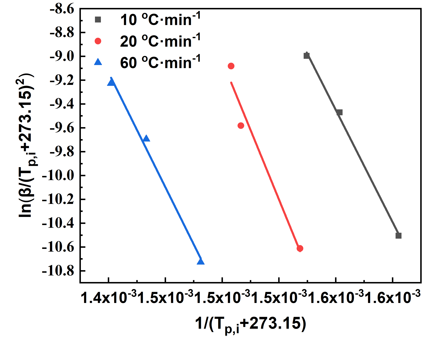Fig. 4. K-S plots at different heating rates

With the Kissinger-Kai method, three peak temperatures were established with the second derivative of TG curves, which corresponded to hemicellulose, cellulose, and lignin at different heating rates. With the peak temperature of each component,was plotted and fit againstas shown in Fig. 4. Additionally, Eα could be obtained with Eq. 11, which was 156.5 kJ/mol for hemicellulose, 193.8 kJ/mol for cellulose, and 159.7 kJ/mol for lignin. Table 2 shows the comparison between the calculated results and the values reported in the literature (Branca et al. 2005; Zhang et al. 2014). Hemicellulose and lignin started to decompose at almost the same time. The decomposition temperature range of lignin was from beginning to end of decomposition, but the maximum decomposition rate occurred in the higher temperature region. The decomposition temperature range of cellulose was narrow, and decomposition rate was high (Chen et al. 2018).

Table 2. Calculation Results of Kinetic Parameters for Hemicellulose, Cellulose, and LigninThe results are generally consistent with the existing models (Branca et al. 2005; Zhang et al. 2014), indicating that the mathematical assumptions used in the Kissinger-Kai method are feasible. The pyrolysis reaction of lignocellulosic biomass is usually three-component parallel reaction. Therefore, pyrolysis temperature range and peak temperatures of the three components of different lignocellulosic biomass are basically the same, and the total activation energy of lignocellulosic biomass will be different due to the different content of each component. The calculation results and calculation methods can be extended to lignocellulosic biomass with different chemical compositions.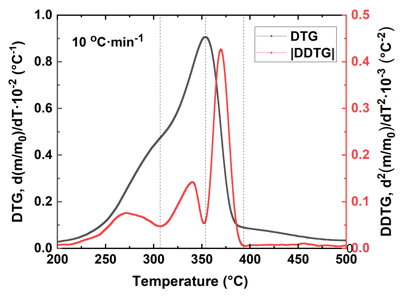Fig. 5. Estimation of peak locations of hemicellulose, cellulose, and lignin

With the Kissinger-Kai method, peak temperatures of different components are shown in Fig. 5. The pyrolysis temperature range of different components with a heating rate of 10 °C could be estimated as follows: from 225 °C to 325 °C for hemicellulose and from 325 °C to 375 °C for cellulose, while lignin was pyrolyzed during the whole decomposition process from 250 °C to 500 °C, which agrees with Ding et al. (2019).

Activation Energies of Pinus sylvestris

The TG records were used to calculate Pinus sylvestris chemical reaction kinetic parameters with KAS and FWO methods, as described earlier. Table 3 shows the reaction temperatures of different heating rates as well as the conversion rate.

Table 3. Reaction Temperature of Different Heating Rates and Conversion Rate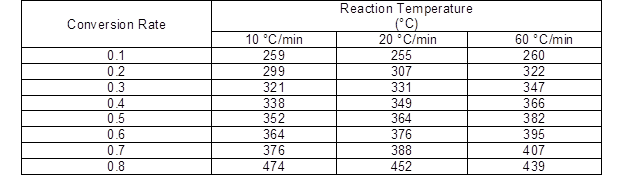There was little or no correlation when conversion values were below 0.1 and above 0.8 (Müsellim et al. 2018). Therefore, the conversion value was chosen between 0.1 and 0.8 for the three heating rates (10 °C/min, 20 °C/min, and 60 °C/min). Using Eq. 8 and Eq. 9,was plotted and fitted against (1/T) with the KAS method, and ln was plotted and fitted against (1/T) with the FWO method, as shown in Figs. 6 and 7, respectively.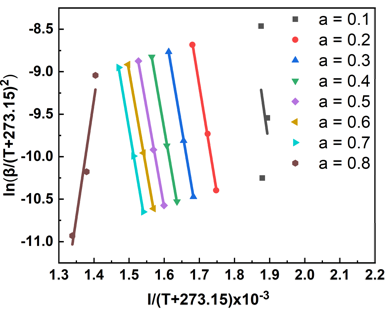Fig. 6. KAS plots for different conversion rates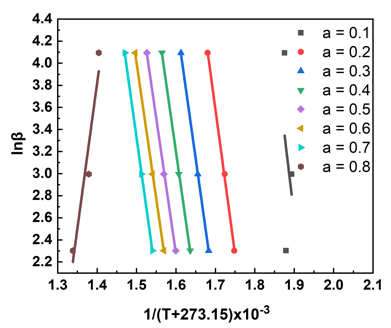Fig. 7. FWO plots for different conversion rates

The straight-line slopes (Eá/R from KAS and 1.052 Eá/R from FWO) were used to calculate Eα at different conversion rates (Damartzis et al. 2011; Arenas et al. 2019). The mean values of Eá were 206.29 kJ/mol and 205.85 kJ/mol with the KAS and FWO methods, respectively, as shown in Table 4. The difference in Eα calculated by KAS and FWO models was less than 2% deviation. The difference approximation of the two methods resulted in the deviation.

Table 4. Calculation Results of Kinetic Parameters and Correlation Factors (R2) for Different Conversion Values by KAS and FWO Models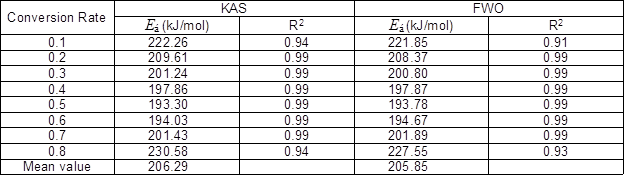Overall, fluctuation in Eα with the increase in conversion rate showed that complex chemical reactions took place in the pyrolysis of Pinus sylvestris. The Eα represents the minimum energy required for a chemical reaction. As Eα increased, the reaction rate decreased, and it became more difficult for the reaction to occur. After water evaporation, the pyrolysis process products of Pinus sylvestris were mainly composed of hemicellulose, cellulose, and lignin. According to the difference in thermal stability for these three components, the distribution of Eα was divided into three stages: the hemicellulose pyrolysis stage when 0.1 < α < 0.3, the cellulose pyrolysis stage when 0.3 < α< 0.7, and the lignin pyrolysis stage when 0.7 < α < 0.8.

In the first stage of 0.1 < α< 0.3, Eα ranged from 222.3 kJ/mol to 201.2 kJ/mol. The Eα started at a higher value and then decreased. This stage mainly included degradation of hemicellulose. Di Blasi and Lanzetta (1997) demonstrated that there are two stages of hemicellulose pyrolysis. The first stage is the break of the branch chain at low temperature, which results in volatile products and intermediate products. The degree of polymerization is decreased. Then, main chains of intermediate products undergo depolymerization reactions to produce various small molecular compounds, such as acetone, formic acid, and propanal, while the temperature increases. Therefore, Eα required for the initial reaction was high, while Eα required for the pyrolysis reaction was low after formation of intermediate products.

The second stage was 0.3 < α< 0.7, and Eα ranged from 197.9 to 194.0 kJ/mol. The Eα value slowly decreased. This stage mainly included degradation of cellulose. Bradbury et al. (1979) noted that cellulose pyrolysis first produces active cellulose as an intermediate product, with a reduced degree of polymerization and molecular chain length. The active cellulose began to degrade with the increase in temperature. However, as degree of polymerization decreased, thermal degradation became more likely to occur; thus, Eα decreased compared with the initial stage.

The third stage was when 0.7 < α < 0.8, and Eα ranged from 201.4 kJ/mol to 230.6 kJ/mol. The Eα increased rapidly, and this stage had a higher Eα. This stage mainly included degradation of lignin because lignin was mainly composed of three phenylpropane structures, which were closely bound together. The thermal degradation was more difficult, and the required activation energy was high. When the conversion rate reached 0.8, Eα rapidly increased, which might have been because coke content of the solid pyrolysis product on the lignin surface increased. Reactivity of carbon was low, but with the increase in temperature, thermal degradation mainly depended on the diffusion reaction zone diffusion rate. With the decrease in degradable substances (volatiles), reaction rate decreased sharply, which resulted in the rapid increase in Eα (Chen et al. 2013; Ma et al. 2015).

CONCLUSIONS

1. The thermogravimetric–differential scanning calorimetry (TG-DSC) curves confirmed that the degradation peak shifted towards a higher region without damaging the decomposition behavior with increasing heating rate. The peak pyrolysis temperatures of the three major components (hemicellulose, cellulose, and lignin) were predicted by the Kissinger-Kai method, and Eα values were calculated to be 156.5, 193.8, and 159.7 kJ/mol, respectively.
2. The Eα values of Pinus sylvestris were estimated by two model-free methods at different conversion rates, which were from 193.3 kJ/mol to 222.3 kJ/mol by the KAS method and from 193.8 to 227.6 kJ/mol by the FWO method. The variation in Ea showed that there were multistep kinetics during the degradation process. The decomposition reaction of hemicellulose, cellulose, and lignin at different temperatures was the main reason for fluctuation in activation energy.
3. At higher heating rates, there was not sufficient time for heat transfer compared to the amount of time at lower heating rates. Therefore, there were temperature gradients in the samples, and maximum exothermic peaks switched to a temperature higher than the maximum pyrolysis temperature (DTG).

ACKNOWLEDGMENTS

This project was supported by the National Natural Science Foundation of China (Grant No. 51805166), Key R & D Plan of Sichuan Science and Technology Program (Grant No. 2020YFN0016), and the China Postdoctoral Science Foundation (Grant No. 2019TQ0084), which are all gratefully acknowledged.

REFERENCES CITED

Ahmad, M. S., Mehmood, M. A., Al-Ayed, O. S., Ye, G., Luo, H., Ibrahim, M., Rashid, U., Nehdi, I. A., and Qadir, G. (2017). “Kinetic analyses and pyrolytic behavior of para grass (Urochloa mutica) for its bioenergy potential,” Bioresource Technol. 224, 708-713. DOI: 10.1016/j.biortech.2016.10.090

Akahira, T., and Sunose, T. (1971). “Joint convention of four electrical institutes,” Res. Rep. Chiba Inst. Technol. 16, 22-31.

Arenas, C. N., Navarro, M. N., and Martínez, J. D. (2019). “Pyrolysis kinetics of biomass wastes using isoconversional methods and the distributed activation energy model,” Bioresource Technol. 288, Article ID 121485. DOI: 10.1016/j.biortech.2019.121485

Bradbury, A. G. W., Sakai, Y., and Shafizadeh, F. (1979). “A kinetic model for pyrolysis of cellulose,” J. Appl. Polym. Sci. 23(11), 3271-3280. DOI: 10.1002/app.1979.070231112

Branca, C., Albano, A., and Di Blasi, C. (2005). “Critical evaluation of global mechanisms of wood devolatilization,” Thermochim. Acta 429(2), 133-141. DOI: 10.1016/j.tca.2005.02.030

Cai, H., Liu, J., Xie, W., Kuo, J., Buyukada, M., and Evrendilek, F. (2019). “Pyrolytic kinetics, reaction mechanisms and products of waste tea via TG-FTIR and Py-GC/MS,” Energy Convers. Manage. 184, 436-447. DOI: 10.1016/j.enconman.2019.01.031

Cai, J., Xu, D., Dong, Z., Yu, X., Yang, Y., Banks, S. W., and Bridgwater, A. V. (2018). “Processing thermogravimetric analysis data for isoconversional kinetic analysis of lignocellulosic biomass pyrolysis: Case study of corn stalk,” Renew. Sust. Energ. Rev. 82, 2705-2715. DOI: 10.1016/j.rser.2017.09.113

Channiwala, S. A., and Parikh, P. P. (2002). “A unified correlation for estimating HHV of solid, liquid and gaseous fuels,” Fuel 81(8), 1051-1063. DOI: 10.1016/S0016-2361(01)00131-4

Chen, D., Gao, A., Cen, K., Zhang, J., Cao, X., and Ma, Z. (2018). “Investigation of biomass torrefaction based on three major components: Hemicellulose, cellulose, and lignin,” Energy Convers. Manage. 169, 228-237. DOI: 10.1016/j.enconman.2018.05.063

Chen, D., Zheng, Y., and Zhu, X. (2013). “In-depth investigation on the pyrolysis kinetics of raw biomass. Part I: Kinetic analysis for the drying and devolatilization stages,” Bioresource Technol. 131, 40-46. DOI: 10.1016/j.biortech.2012.12.136

Chen, J., He, Y., Liu, J., Liu, C., Xie, W., Kuo, J., Zhang, X., Li, S., Liang, J., Sun, S., et al. (2019). “The mixture of sewage sludge and biomass waste as solid biofuels: Process characteristic and environmental implication,” Renew. Energ. 139, 707-717. DOI: 10.1016/j.renene.2019.01.119

Damartzis, T., Vamvuka, D., Sfakiotakis, S., and Zabaniotou, A. (2011). “Thermal degradation studies and kinetic modeling of cardoon (Cynara cardunculus) pyrolysis using thermogravimetric analysis (TGA),” Bioresource Technol. 102(10), 6230-6238. DOI: 10.1016/j.biortech.2011.02.060

Dhyani, V., Kumar, J., and Bhaskar, T. (2017). “Thermal decomposition kinetics of sorghum straw via thermogravimetric analysis,” Bioresource Technol. 245, 1122-1129. DOI: 10.1016/j.biortech.2017.08.189

Di Blasi, C., and Lanzetta, M. (1997). “Intrinsic kinetics of isothermal xylan degradation in inert atmosphere,” J. Anal. Appl. Pyrol. 40-41, 287-303. DOI: 10.1016/s0165-2370(97)00028-4

Ding, Y., Ezekoye, O. A., Lu, S., and Wang, C. (2016). “Thermal degradation of beech wood with thermogravimetry/Fourier transform infrared analysis,” Energy Convers. Manage. 120, 370-377. DOI: 10.1016/j.enconman.2016.05.007

Ding, Y., Wang, C., Chaos, M., Chen, R., and Lu, S. (2016). “Estimation of beech pyrolysis kinetic parameters by shuffled complex evolution,” Bioresource Technol. 200, 658-665. DOI: 10.1016/j.biortech.2015.10.082

Ding, Y., Zhang, Y., Zhang, J., Zhu, R., Ren, Z., Guo and H. (2019). “Kinetic parameters
estimation of pinus sylvestris pyrolysis by Kissinger-Kai method coupled with Particle Swarm Optimization and global sensitivity analysis,” Bioresour. Technol. 293. DOI: 10.1016/j.biortech.2019.122079

Ding, Y., kazui, F., Ofodike a., E., Lu, S., Wang, C. and Li, C. (2020). “Experimental and numerical simulation of multi-component combustion of typical charring material,” Combustion and Flame 211, 417-429. DOI:10.1016/j.combustflame.2019.10.016

Fermoso, J., and Mašek, O. (2018). “Thermochemical decomposition of coffee ground residues by TG-MS: A kinetic study,” J. Anal. Appl. Pyrol. 130, 358-367. DOI: 10.1016/j.jaap.2017.12.007

Font, R., Fullana, A., and Conesa, J. (2005). “Kinetic models for the pyrolysis and combustion of two types of sewage sludge,” J. Anal. Appl. Pyrol. 74(1-2), 429-438. DOI: 10.1016/j.jaap.2004.10.009

Grønli, M. G., Várhegyi, G., and Di Blasi, C. (2002). “Thermogravimetric analysis and devolatilization kinetics of wood,” Ind. Eng. Chem. Res. 41(17), 4201-4208. DOI: 10.1021/ie0201157

Huang, J., Liu, J., Chen, J., Xie, W., Kuo, J., Lu, X., Chang, K., Wen, S., Sun, G., Cai, H., et al. (2018). “Combustion behaviors of spent mushroom substrate using TG-MS and TG/FTIR: Thermal conversion, kinetic, thermodynamic and emission analyses,” Bioresource Technol. 266, 389-397. DOI: 10.1016/j.biortech.2018.06.106

Huang, J., Zhang, J., Liu, J., Xie, W., Kuo, J., Chang, K., Buyukada, M., Evrendilek, F., and Sun, S. (2019). “Thermal conversion behaviors and products of spent mushroom substrate in CO2 and N2 atmospheres: Kinetic, thermodynamic, TG and Py-GC/MS analyses,” J. Anal. Appl. Pyrol. 139, 177-186. DOI: 10.1016/j.jaap.2019.02.002

Jiang, L., Xiao, H. H., He, J. J., Sun, Q., Gong, L., and Sun, J. H. (2015). “Application of genetic algorithm to pyrolysis of typical polymers,” Fuel Process. Technol. 138, 48-55. DOI: 10.1016/j.fuproc.2015.001

Kissinger, H. E. (1956). “Variation of peak temperature with heating rate in differential thermal analysis,” J. Res. Natl. Bur. Stand. 57(4), 217-221.

Kissinger, H. E. (1957). “Reaction kinetics in differential thermal analysis,” Anal. Chem. 29(11), 1702-1706. DOI: 10.1021/ac60131a045

Lahijani, P., Mohammadi, M., and Mohamed, A. R. (2019). “Investigation of synergism and kinetic analysis during CO2 co-gasification of scrap tire char and agro-wastes,” Renew. Energ. 142, 147-157. DOI: 10.1016/j.renene.2019.04.113

Li, K. Y., Huang, X., Fleischmann, C., Rein, G., and Ji, J. (2014). “Pyrolysis of medium-density fiberboard: Optimized search for kinetics scheme and parameters via a genetic algorithm driven by Kissinger’s method,” Energ. Fuel. 28(9), 6130-6139. DOI: 10.1021/ef501380c

Ma, Z., Chen, D., Gu, J., Bao, B., and Zhang, Q. (2015). “Determination of pyrolysis characteristics and kinetics of palm kernel shell using TGA-FTIR and model-free integral methods,” Energy Convers. Manage. 89, 251-259. DOI: 10.1016/j.enconman.2014.09.074

Müsellim, E., Tahir, M. H., Ahmad, M. S., and Ceylan, S. (2018). “Thermokinetic and TG/DSC-FTIR study of pea waste biomass pyrolysis,” Appl. Therm. Eng. 137, 54-61. DOI: 10.1016/j.applthermaleng.2018.03.050

Nhuchhen, D. R., and Abdul Salam, P. (2012). “Estimation of higher heating value of biomass from proximate analysis: A new approach,” Fuel 99, 55-63. DOI: 10.1016/j.fuel.2012.04.015

Nurek, T., Gendek, A., and Roman, K. (2019). “Forest residues as a renewable source of energy: Elemental composition and physical properties,” BioResources 14(1), 6-20. DOI: 10.15376/biores.14.1.6-20

Rodríguez-Jiménez, S., Duarte-Aranda, S., and Canché-Escamilla, G. (2019). “Chemical composition and thermal properties of tropical wood from the Yucatán dry forests,” BioResources 14(2), 2651-2666. DOI: 10.15376/biores.14.2.2651-2666

Shi, S., Zhou, X., Chen, W., Wang, X., Nguyen, T., and Chen, M. (2017). “Thermal and kinetic behaviors of fallen leaves and waste tires using thermogravimetric analysis,” BioResources 12(3), 4707-4721. DOI: 10.15376/biores.12.3.4707-4721

Vyazovkin, S., Burnham, A. K., Criado, J. M., Pérez-Maqueda, L. A., Popescu, C., and Sbirrazzuoli, N. (2011). “ICTAC kinetics committee recommendations for performing kinetic computations on thermal analysis data,” Thermochim. Acta 520(1-2), 1-19. DOI: 10.1016/j.tca.2011.03.034

Wang, S., Dai, G., Yang, H., and Luo, Z. (2017). “Lignocellulosic biomass pyrolysis mechanism: A state-of-the-art review,” Prog. Energ. Combust. Sci. 62, 33-86. DOI: 10.1016/j.pecs.2017.05.004

Wen, S., Yan, Y., Liu, J., Buyukada, M., and Evrendilek, F. (2019). “Pyrolysis performance, kinetic, thermodynamic, product and joint optimization analyses of incense sticks in N2 and CO2 atmospheres,” Renew. Energ. 141, 814-827. DOI: 10.1016/j.renene.2019.04.040

Wu, Z., Zhang, B., Hu, Q., Hao, W., Ma, C., Li, Y., and Yang, B. (2019). “Products distribution and kinetic analysis on gaseous products during fast pyrolysis of two kinds of biomass pellet,” Fuel 249, 8-14. DOI: 10.1016/j.fuel.2019.03.100

Zhang, J., Chen, T., Wu, J., and Wu, J. (2014). “A novel Gaussian-DAEM-reaction model for the pyrolysis of cellulose, hemicellulose and lignin,” RSC Adv. 4(34), 17513-17520. DOI: 10.1039/c4ra01445f

Zhang, J., Liu, J., Evrendilek, F., Zhang, X., and Buyukada, M. (2019). “TG-FTIR and Py-GC/MS analyses of pyrolysis behaviors and products of cattle manure in CO2 and N2 atmospheres: Kinetic, thermodynamic and machine-learning models,” Energy Convers. Manage. 195, 346-359. DOI: 10.1016/j.enconman.2019.05.019

Zou, H., Evrendilek, F., Liu, J., and Buyukada, M. (2019). “Combustion behaviors of pileus and stipe parts of Lentinus edodes using thermogravimetric-mass spectrometry and Fourier transform infrared spectroscopy analyses: Thermal conversion, kinetic, thermodynamic, gas emission and optimization analyses,” Bioresource Technol. 288, 121481. DOI: 10.1016/j.biortech.2019.121481

Article submitted: December 12, 2019; Peer review completed: May 16, 2020; Revised version received and accepted: May 25, 2020; Published: May 29, 2020.

DOI: 10.15376/biores.15.3.5577-5592